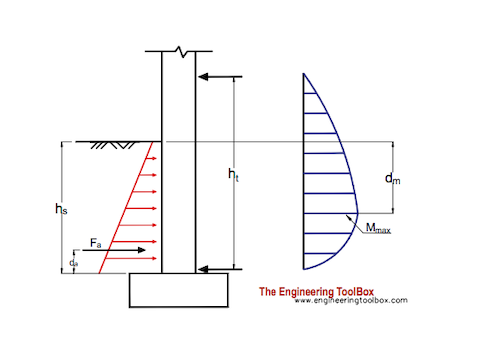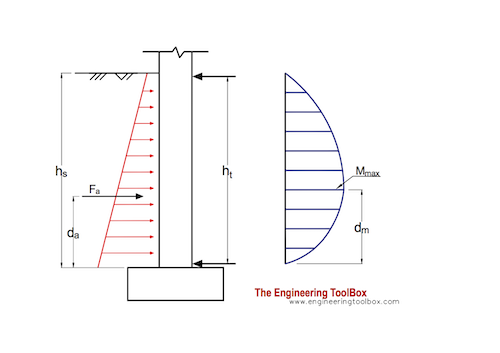Engineering ToolBox - Resources, Tools and Basic Information for Engineering and Design of Technical Applications!

# Earth Pressure Acting on Basement Walls

## Calculate lateral earth pressure acting on basement walls.

### 1st Floor above Ground Level

The forces that keeps the basement wall in position - the force at the basement floor/slab and the force from the 1. floor joists above ground level - are indicated in the figure below.The resultant force due to the earth pressure acting on a basement wall can be calculated as

Fa = 0.5 K γ hs2                             (1a)

where

Fa = resultant force acting on the basement wall (kN)

hs = height of backfill soil (m)

γ = specific weight of backfill soil (kN/m3)

K = coefficient of earth pressure at rest

Coefficient of earth pressure at rest can be calculated as

K = μ / (1 - μ)                      (1b)

where

μ = Poisson's ratio

Poisson's ratios for some typical backfill soils:

• clay: 0.41
• sand : 0.29
• sandy clay: 0.37
• sandy loam: 0.31

Specific weight can be calculated as

γ = ρ ag                               (1c)

where

ρ = density of soil (kg/m3)

ag = acceleration of gravity (9.81 m/s2)

Density for some typical backfill materials:

• clay, dry: 1600 kg/m3
• sand, dry: 1555 kg/m3

The acting position of the acting force can be calculated as

da = hs / 3                           (1d)

where

da = distance from the bottom of the basement wall    (m)

The maximum bending moment acting in the basement wall can be calculated as

Mmax = (Fa hs / 3 ht) (hs + (2 hs / 3)(hs / (3 ht))1/2)                            (1e)

where

Mmax = maximum moment in the basement wall (Nm)

The position of the max moment in the basement wall can be calculated as

dm = hs (hs / (3 ht))1/2                 (1f)

Note! - cracking of a basement wall is likely to occur where the moment is at the maximum. Due to tension reinforcement bars should be concentrated closer to the inside wall.

### 1st Floor at Ground Level

The forces that keeps the basement wall in position - the force at the basement floor/slab and the force from the 1. floor joists at ground level - are indicated in the figure below.The resultant force due to the earth pressure acting on a basement wall can be calculated as

Fa = 0.5 K γ hs2                             (2a)

The acting position of the acting force can be calculated as

da = hs / 3                           (2b)

where

da = distance from the bottom of the basement wall    (m)

The maximum moment acting in the basement wall can be calculated as

Mmax = 0.128 Fa hs                                (2c)

where

Mmax = maximum moment in the basement wall (Nm)

The position of the max. moment in the basement wall can be calculated as

dm = 0.42 hs                   (2e)

#### Example - Earth Pressure on Basement Wall

A basement wall with height 2.5 m is backfilled with sand.

The Poisson's ratio for sand is 0.29 and the coefficient of earth pressure can be calculated as

K = 0.29 / (1 - 0.29)

= 0.41

The specific weight for the sand can be calculated as

γ = (1555 kg/m3) (9.81 m/s2)

= 15255 N/m3

= 15.3 kN/m3

The resultant force acting on the basement wall can be calculated as

Fa = 0.5 (0.41) (15.3 kN/m3) (2.5 m)2

= 19.6 kN

The acting position of the resultant force can be calculated as

da = (2.5 m) / 3

= 0.83 m

The maximum moment acting in the basement wall can be calculated as

Mmax = 0.128 (19.6 kN) (2.5 m)

= 6.3 kNm

The position of the max. moment in the basement wall can be calculated as

dm = 0.42 (2.5 m)

= 1.05

## Related Topics

• Statics - Loads - forces and torque, beams and columns.

## Engineering ToolBox - SketchUp Extension - Online 3D modeling!

Add standard and customized parametric components - like flange beams, lumbers, piping, stairs and more - to your Sketchup model with the Engineering ToolBox - SketchUp Extension - enabled for use with the amazing, fun and free SketchUp Make and SketchUp Pro .Add the Engineering ToolBox extension to your SketchUp from the SketchUp Pro Sketchup Extension Warehouse!

Translate

## Privacy

We don't collect information from our users. Only emails and answers are saved in our archive. Cookies are only used in the browser to improve user experience.

Some of our calculators and applications let you save application data to your local computer. These applications will - due to browser restrictions - send data between your browser and our server. We don't save this data.

## Citation

• Engineering ToolBox, (2003). Earth Pressure Acting on Basement Walls. [online] Available at: https://www.engineeringtoolbox.com/basement-wall-earth-pressure-d_558.html [Accessed Day Mo. Year].

Modify access date.

. .

#### Scientific Online Calculator3 30